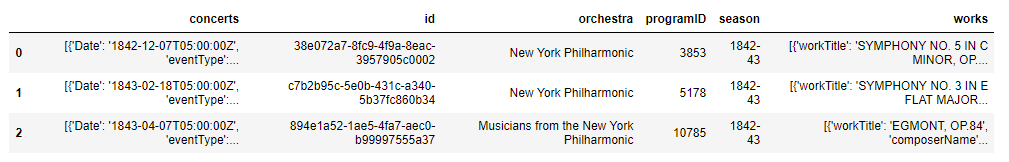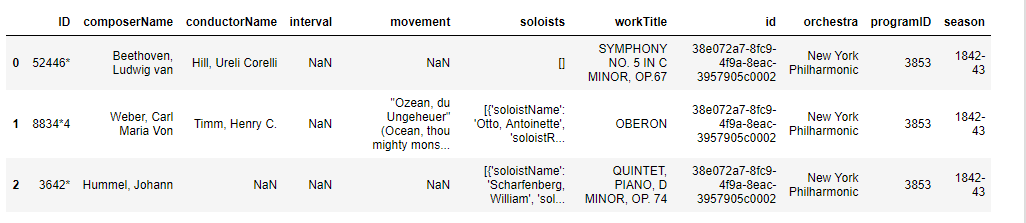# Pandas | Parsing JSON Dataset

Parsing of JSON Dataset using pandas is much more convenient. Pandas allow you to convert a list of lists into a Dataframe and specify the column names separately.

A JSON parser transforms a JSON text into another representation must accept all texts that conform to the JSON grammar. It may accept non-JSON forms or extensions. An implementation may set the following:

• limits on the size of texts that it accepts,
• limits on the maximum depth of nesting,
• limits on the range and precision of numbers,
• set limits on the length and character contents of strings.

Working with large JSON datasets can be deteriorating, particularly when they are too large to fit into memory. In cases like this, a combination of command line tools and Python can make for an efficient way to explore and analyze the data.

Importing JSON Files:

Manipulating the JSON is done using the Python Data Analysis Library, called pandas.

`import pandas as pd`

Now you can read the JSON and save it as a pandas data structure, using the command `read_json`.

pandas.read_json (path_or_buf=None, orient = None, typ=’frame’, dtype=True, convert_axes=True, convert_dates=True, keep_default_dates=True, numpy=False, precise_float=False, date_unit=None, encoding=None, lines=False, chunksize=None, compression=’infer’)

 `import` `pandas as pd ` `# Creating Dataframe  ` `df ``=` `pd.DataFrame([[``'a'``, ``'b'``], [``'c'``, ``'d'``]], ` `                  ``index ``=``[``'row 1'``, ``'row 2'``], ` `                  ``columns ``=``[``'col 1'``, ``'col 2'``]) ` ` `  `# Indication of expected JSON string format ` `print``(df.to_json(orient ``=``'split'``)) ` ` `  `print``(df.to_json(orient ``=``'index'``)) `

Output:

```{"columns":["col 1", "col 2"],
"index":["row 1", "row 2"],
"data":[["a", "b"], ["c", "d"]]}

{"row 1":{"col 1":"a", "col 2":"b"},
"row 2":{"col 1":"c", "col 2":"d"}}
```

Convert the object to a JSON string using dataframe.to_json:

DataFrame.to_json(path_or_buf=None, orient=None, date_format=None, double_precision=10, force_ascii=True, date_unit=’ms’, default_handler=None, lines=False, compression=’infer’, index=True)

Read the JSON File directly from Dataset:

 `import` `pandas as pd ` ` `  `data ``=` `pd.read_json(``'http://api.population.io/1.0/population/India/today-and-tomorrow/?format = json'``) ` `print``(data) `

Output:

```total_population
0  {'date': '2019-03-18', 'population': 1369169250}
1  {'date': '2019-03-19', 'population': 1369211502}
```

Nested JSON Parsing with Pandas:

Nested JSON files can be time consuming and difficult process to flatten and load into Pandas.
We are using nested ”’raw_nyc_phil.json.”’ to create a flattened pandas data frame from one nested array then unpack a deeply nested array.

Code #1:
Let’s unpack the works column into a standalone dataframe. We’ll also grab the flat columns.

 `import` `json  ` `import` `pandas as pd  ` `from` `pandas.io.json ``import` `json_normalize  ` ` `  `with ``open``(``'https://github.com/a9k00r/python-test/blob/master/raw_nyc_phil.json'``) as f: ` `    ``d ``=` `json.load(f) ` ` `  `# lets put the data into a pandas df ` `# clicking on raw_nyc_phil.json under "Input Files" ` `# tells us parent node is 'programs' ` `nycphil ``=` `json_normalize(d[``'programs'``]) ` `nycphil.head(``3``) `

Output:Code #2:
Let’s unpack the works column into a standalone dataframe using json_normaliz.

 `works_data ``=` `json_normalize(data ``=` `d[``'programs'``], ` `                            ``record_path ``=``'works'``,  ` `                            ``meta ``=``[``'id'``, ``'orchestra'``, ``'programID'``, ``'season'``]) ` `works_data.head(``3``) `

Output:Code #3:

Let’s flatten the ‘soloists’ data here by passing a list. Since soloists is nested in work.

 `soloist_data ``=` `json_normalize(data ``=` `d[``'programs'``], ` `                              ``record_path ``=``[``'works'``, ``'soloists'``], ` `                              ``meta ``=``[``'id'``]) ` ` `  `soloist_data.head(``3``) `

Output:My Personal Notes arrow_drop_upCheck out this Author's contributed articles.

If you like GeeksforGeeks and would like to contribute, you can also write an article using contribute.geeksforgeeks.org or mail your article to contribute@geeksforgeeks.org. See your article appearing on the GeeksforGeeks main page and help other Geeks.

Please Improve this article if you find anything incorrect by clicking on the "Improve Article" button below.

Article Tags :

2

Please write to us at contribute@geeksforgeeks.org to report any issue with the above content.